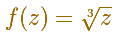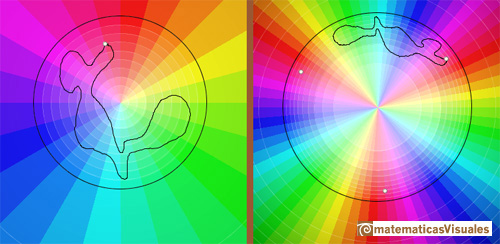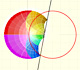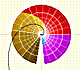The usual definition of a function is restrictive. We may broaden the definition of a function to allow f(z) to have many differente values for a single value of z. In this case f is called a "many-valued function" or a multifunction.

For example, we know thathas three different values (if z is not zero), so it is a three-valued multifunction.

In this case, we see that each color is shown three times and that each z value has three different images with the same module but different argument.If we arbitrarily choose one of the three images of a given point z and gradually moves away from this initial position then the image moves away in a completely determined way -its distance from the origin is the cube root of the distance of z, and its angular speed is one third that of z. (Needham, p.91)

As z travels along a closed loop like in this picture, its image f(z) travels along a closed loop and returns to its original value.However, if z instead travels along a closed loop which goes round the origin once, then f(z) does not return to its original value but instead ends up at a different cube root. Note that the detailed shape of the loop is irrelevant, all that matters is that it encircles the origin once. (Needham, p.91)Similarly, if z travels along a closed loop encircling the origin twice, then f(z) ends up at the third and final cube root. (Needham, p.91)Clearly, if z travels along a loop that encircled the origin three times, then f(z) returns to its original root. (Needham, p.91)The point z = 0 is called a branch point of the multifunction f(z).

REFERENCES

Tristan Needham - Visual Complex Analysis. (pag. 91) - Oxford University PressMultifunctions can have more than one branch point. In this page we can see a two-valued multifunction with two branch points.Inversion is a plane transformation that transform straight lines and circles in straight lines and circles.Una primera aproximación a estas transformaciones. Representación de dos haces coaxiales de circunferencias ortogonales.The Complex Exponential Function extends the Real Exponential Function to the complex plane.The Complex Cosine Function extends the Real Cosine Function to the complex plane. It is a periodic function that shares several properties with his real ancestor.The Complex Cosine Function maps horizontal lines to confocal ellipses.Examples of complex functions: polynomials, rationals and Moebius Transformations.